# Numb3rs 508: Thirty Six Hours

In this episode, a train crash occurs causing fatalities and a leak of toxic chemicals. It's up to the FBI team to help rescue the living victims as well as figure out the cause of the crash. Math and physics play a role in both of these tasks.

In order to help rescue the living victims in one of the rail cars, Charlie uses small robotic cars known as swarm bots to survey the situation and map out a route for the rescue team. To determine the cause of the crash, Charlie utilizes some basic physics to deduce that the conductor was lied to about the mass of the train and cargo.

In this website, we'll focus on some of the math and physics that Charlie needs in order to figure out the cause of the crash. However, if you wish to learn about swarm robotics, the following are a few places to begin.

1. http://www.swarm-robotics.org/
2. http://www.swarm-bots.org/
3. http://en.wikipedia.org/wiki/Swarm_robotics

## Some Physics

### Linear Motion

Suppose an object is moving along a straight line. We can fix a reference point and graph the position of the object (relative to this reference point) over time. Let s(t) denote the function which has this graph. The value of s at a given time t* is the displacement of the object at time t*. For example, if s(t*)=5 then the object is 5 units in front of the reference point at time t*. If s(t*)= -5, then, at time t*, the object is 5 units behind the reference point. Therefore, if t1 and t2 (with t1 < t2) are two times, we have that the average velocity of the object between time t1 and t2 is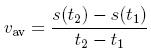If Δt=t2-t1, we can rewrite the above equation asFor those of you that are familiar with basic calculus, notice that the formula for average velocity would look like the definition of a derivative if there were a limit thrown in. In fact, if we take the limit as Δt goes to 0, we obtain instantaneous velocity at time t1. That is,We have that s(t) is displacement, s'(t)=v(t) is velocity and v'(t)=a(t) is acceleration. But what happens if we take another derivative? What physical quantity does a'(t) represent? The answer is jerk. You can definitely feel this when riding in a car that quickly changes how much it's accelerating!
As indicated above, the velocity function is denoted by v(t).

Analogously, we can define average acceleration (over time Δt) and instantaneous acceleration (at time t) by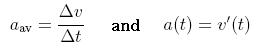respectively.

Let's consider the example s(t) = -(t-3)2 + 9. This describes the motion of an object which is at the reference point at t=0, then moves forward, changes direction and eventually ends up behind the reference point. We also have that v(t)=-2(t-3) and that a(t) = -2. That is, the object with position described by the function s(t) has constant acceleration. Below are the graphs of s(t), v(t) and a(t).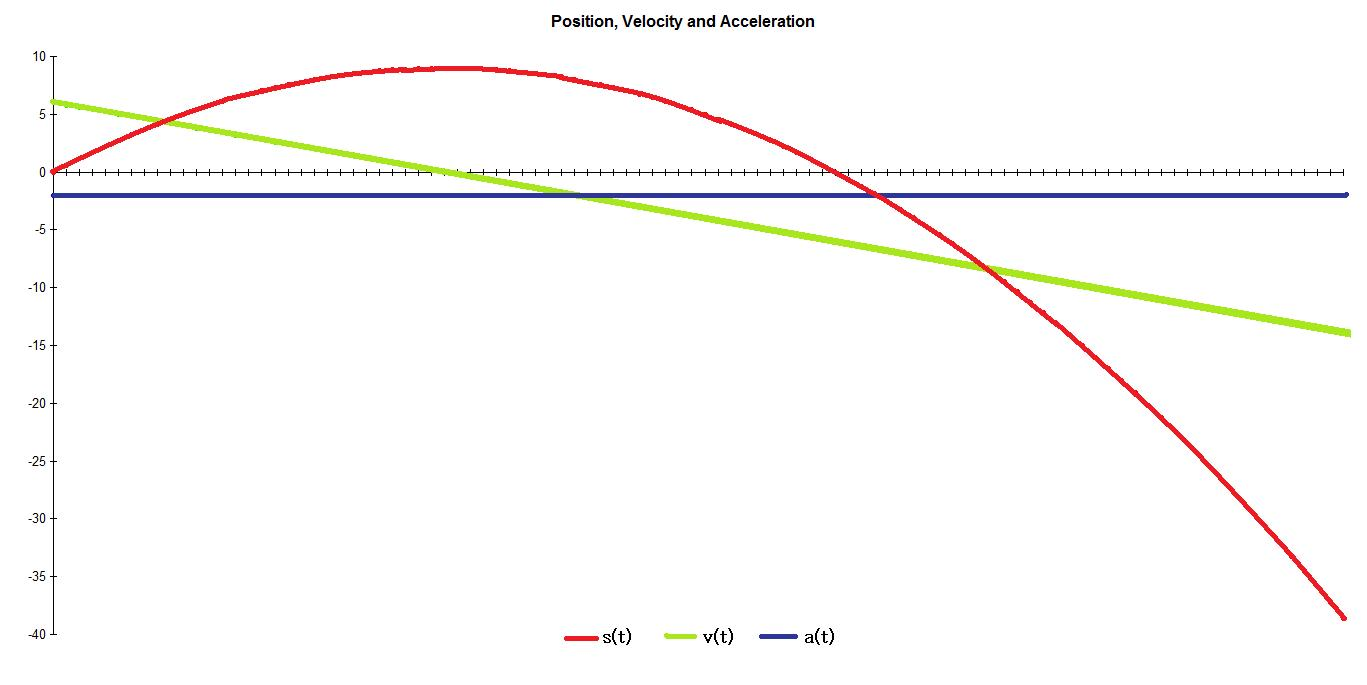Activity 1: Linear Motion
Suppose that an object X is experiencing linear motion. The position of X over time is described by the function s(t) = t2+5t-7.
1. Sketch the graph of s. Describe the motion of X.
2. What are v(t)=s'(t) and a(t)=v'(t)? Sketch the graphs of v and a. Now describe the motion of X in more detail.

#### Constant Acceleration

When acceleration is constant, one can derive equations of (linear) motion by solving the differential equations described above. For example, consider the equation a(t)=v'(t). If acceleration is a constant a then we have the equation a = v'(t). Separating variables and integrating from ti to t yields the equation a⋅(t-ti) = v(t) - v(ti). Thus, if we put Δt = t-ti and vi=v(ti), we have that v(t) = vi+aΔt. Using similar techniques, we can get the following equations of linear motion: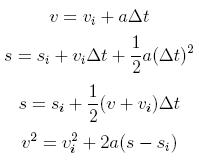In the above equations, vi and v represent initial velocity and current velocity respectively. si and s represent initial position and current position respectively.

One final remark is needed: Be sure to be consistant with units when using the equations of motion! For example, don't measure acceleration in m/s2 and velocity in km/h. Either measure both quantities using meters and seconds or both using kilometers and hours.

### Force and Friction

Force is a vector quantity. That is, like velocity and acceleration above, it has both a magnitude and direction. When a force is applied to an object, it causes the object to accelerate in the direction of the applied force. The relationship between force and acceleration can be made precise with the famous equation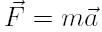Here F is force, m is mass and a is acceleration.

Of course, many forces can act on the same object. It is even possible that the net force (i.e. the vector sum of all of the forces acting on the object) is 0. In this case, the object will stay put. When multiple forces act on an object we have the equation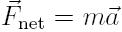A free body diagram is helpful in keeping track of all the forces that act on an object. It is a diagram where the object is represented by a box (or other simple shape) and includes arrows of different lengths and directions to represent the various forces that act on the object. Below is an example of a free body diagram for an object being pushed along a table by an applied force F_a. F_g, F_N and F_f denote force of gravity, normal force and force of friction respectively.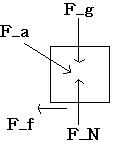The SI unit for force is the newton (N). One newton is equal to one kg⋅m/s2. It is again very important to be careful with units when using the force equations above.

#### Some Common Forces

Gravity: The force due to Earth's gravity always points downwards (towards the center of the Earth). It is given by the equation F = mg where g is approximately equal to 9.81 m/s2.

Normal Force: When an object is in contact with a surface, the normal force on the object is a force that points perpendicular to and out from the surface. It is equal in magnitude to the force that the object exerts on the surface in the exact opposite direction. For example, if a box is resting on a table, the normal force on the box will point upwards and be equal in magnitude to the force of gravity on the box.

Static Friction: This is the force of friction between two objects that are not moving relative to one another. For example, static friction is the force that can prevent a block resting on a sloped surface from sliding down the slope. In order for the object to start to move, the force of static friction must be overcome by an external force (ex: gravity or a push). The frictional force that must be overcome is given by μsFN where μs is the coefficient of static friction and FN is the normal force. Note that the coefficient of friction is a number that depends on the two materials that come into contact.

Kinetic Friction: Kinetic friction is the force of friction between two objects that are moving relative to one another. It is given by μkFN where μk is the coefficient of kinetic friction. Note that the coefficient of kinetic friction is typically smaller than the coefficient of static friction for the same materials.

Activity 2: Sliding Block
A 2kg block is placed on a 30o inclined plane. The coefficient of static friction between the block and the plane is 0.4.
1. Draw a free body diagram and label all forces.
2. Calculate the normal force.
3. Will the block stay put or will it begin to slide down the surface? Explain.
Activity 3: Angle of Friction
Let μ be the coefficient of static friction between a block and an inclined plane. Determine an expression (in terms of μ) for the maximum angle of incline of the plane before the block starts to slide.

### Putting it all together: How do you stop a speeding train?

Activity 4: Hit the brakes!
Imagine that you're the conductor of a train. Your train weighs 600 000 kg and is moving at a speed of 120 km/h. Suddenly, you notice a stalled truck on the tracks 150 m away. You have to hit the brakes. Your train slows down due to kinetic friction, but will it stop in time?
1. Draw a free body diagram of the situation and label all forces.
2. Assume that the coefficient of kinetic friction between the train and tracks is 0.45. Does the train stop in time?
3. Now suppose that you can control exactly how much force is applied to the train. What force must be appied to the train to cause it to stop just in the nick of time?